Share

# HC Verma solutions for Class 12 Concepts of Physics 1 chapter 10 - Rotational Mechanics [Latest edition]

Textbook page

#### Chapters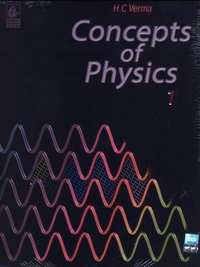## Chapter 10: Rotational Mechanics

### HC Verma solutions for Class 12 Concepts of Physics 1 Chapter 10 Rotational Mechanics Short Answers [Page 192]

Short Answers | Q 1 | Page 192

Can an object be in pure translation as well as in pure rotation?

Short Answers | Q 2 | Page 192

A simple pendulum is a point mass suspended by a light thread from a fixed point. The particle is displaced towards one side and then released. It makes small oscillations. Is the motion of such a simple pendulum a pure rotation? If yes, where is the axis of rotation?

Short Answers | Q 3 | Page 192

In a rotating body, $\alpha = \alpha r\text{ and }\nu = \omega r.$ Thus $\frac{\alpha}{\alpha} = \frac{\nu}{\omega}.$ Can you use the theorems of ration and proportion studied in algebra so as to write $\frac{\alpha + \alpha}{\alpha - \alpha} = \frac{\nu + \omega}{\nu - \omega}$

Short Answers | Q 4 | Page 192

A ball is whirled in a circle by attaching it to a fixed point with a string. Is there an angular rotation of the ball about its centre? If yes, is this angular velocity equal to the angular velocity of the ball about the fixed point?

Short Answers | Q 5 | Page 192

The moon rotates about the earth in such a way that only one hemisphere of the moon faces the earth (see the following figure). Can we ever see the "other face" of the moon from the earth? Can a person on the moon ever see all the faces of the earth?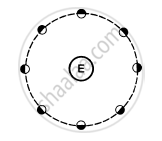Short Answers | Q 6 | Page 192

The torque of the weight of any body about any vertical axis is zero. If it always correct?

Short Answers | Q 7 | Page 192

The torque of a force $\overrightarrow F$ about a point is defined as $\overrightarrow\Gamma = \overrightarrow r \times \overrightarrow F.$ Suppose $\overrightarrow r, \overrightarrow F$ and $\overrightarrow \Gamma$ are all nonzero. Is $r \times \overrightarrow\Gamma || \overrightarrow F$ always true? Is it ever true?

Short Answers | Q 8 | Page 192

A heavy particle of mass m falls freely near the earth's surface. What is the torque acting on this particle about a point 50 cm east to the line of motion? Does this torque produce any angular acceleration in the particle?

Short Answers | Q 9 | Page 192

If several forces act on a particle, the total torque on the particle may be obtained by first finding the resultant force and then taking torque of this resultant. Prove this. Is this result valid for the forces acting on different particles of a body in such a way that their lines of action intersect at a common point?

Short Answers | Q 10 | Page 192

If the sum of all the forces acting on a body is zero, is it necessarily in equilibrium? If the sum of all the forces on a particle is zero, is it necessarily in equilibrium?

Short Answers | Q 11 | Page 192

If the angular momentum of a body is found to be zero about a point, is it necessary that it will also be zero about a different point?

Short Answers | Q 12 | Page 192

If the resultant torque of all the forces acting on a body is zero about a point, is it necessary that it will be zero about any other point?

Short Answers | Q 13 | Page 192

A body is in translational equilibrium under the action of coplanar forces. If the torque of these forces is zero about a point, is it necessary that it will also be zero about any other point?

Short Answers | Q 14 | Page 192

A rectangular brick is kept on a table with a part of its length projecting out. It remains at rest if the length projected is slightly less than half the total length but it falls down if the length projected is slightly more than half the total length. Give reason.

Short Answers | Q 15 | Page 192

When a fat person tries to touch his toes, keeping the legs straight, he generally falls. Explain with reference to the following figure.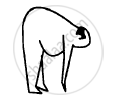Short Answers | Q 16 | Page 192

A ladder is resting with one end on a vertical wall and the other end on a horizontal floor. If it more likely to slip when a man stands near the bottom or near the top?

Short Answers | Q 17 | Page 192

When a body is weighed on an ordinary balance we demand that the arum should be horizontal if the weights on the two pans are equal. Suppose equal weights are put on the two pans, the arm is kept at an angle with the horizontal and released. Is the torque of the two weights about the middle point (point of support) zero? Is the total torque zero? If so, why does the arm rotate and finally become horizontal?

Short Answers | Q 18 | Page 192

The density of a rod AB continuously increases from A to B. Is it easier to set it in rotation by clamping it at A and applying a perpendicular force at B or by clamping it at B and applying the force at A?

Short Answers | Q 19 | Page 192

When tall building are constructed on earth, the duration of day-night slightly increases. Is it true?

Short Answers | Q 20 | Page 192

If the ice at the poles melts and flows towards the equator, how will it affect the duration of day-night?

Short Answers | Q 21 | Page 192

A hollow sphere, a solid sphere, a disc and a ring all having same mass and radius are rolled down on an inclined plane. If no slipping takes place, which one will take the smallest time to cover a given length?

Short Answers | Q 22 | Page 192

A sphere rolls on a horizontal surface. If there any point of the sphere which has a vertical velocity?

### HC Verma solutions for Class 12 Concepts of Physics 1 Chapter 10 Rotational Mechanics MCQ [Pages 193 - 194]

MCQ | Q 1 | Page 193

Let $\overrightarrow A$ be a unit vector along the axis of rotation of a purely rotating body and $\overrightarrow B$ be a unit vector along the velocity of a particle P of the body away from the axis. The value of $\overrightarrow A.\overrightarrow B$ is ____________ .

• 1

• -1

• 0

• None of these

MCQ | Q 2 | Page 193

A body is uniformly rotating about an axis fixed in an inertial frame of reference. Let $\overrightarrow A$ be a unit vector along the axis of rotation and $\overrightarrow B$ be the unit vector along the resultant force on a particle P of the body away from the axis. The value of $\overrightarrow A.\overrightarrow B$ is _________.

• 1

• -1

• 0

• None of these

MCQ | Q 3 | Page 193

A particle moves with a constant velocity parallel to the X-axis. Its angular momentum with respect to the origin ____________.

• is zero

• remains constant

• goes on increasing

• goes on decreasing

MCQ | Q 4 | Page 193

A body is in pure rotation. The linear speed $\nu$ of a particle, the distance r of the particle from the axis and the angular velocity $\omega$ of the body are related as $\omega = \frac{\nu}{r}.$ Thus

• $\omega = \frac{\nu}{r}$

• $\omega \propto r$

• $\omega = 0$

• $\omega$ is independent of r

MCQ | Q 5 | Page 193

The following figure shows a small wheel fixed coaxially on a bigger one of double the radius. The system rotates about the common axis. The strings supporting A and B do not slip on the wheels. If x and y be the distance travelled by A and B in the same time interval, then _________ .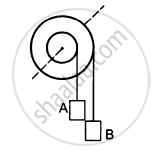• x = 2y

• x = y

• y = 2x

• None of these

MCQ | Q 6 | Page 193

A body is rotating uniformly about a vertical axis fixed in an inertial frame. The resultant force on a particle of the body not on the axis is _________ .

• vertical

• horizontal and skew with the axis

• horizontal and intersecting the axis

• None of these

MCQ | Q 7 | Page 193

A body is rotating nonuniformity about a vertical axis fixed in an inertial frame. The resultant force on a particle of the body not on the axis is _________ .

• vertical

• horizontal and skew with the axis

• horizontal and intersecting the axis

• None of these

MCQ | Q 8 | Page 193

Let $\overrightarrow F$ be a force acting on a particle having position vector $\overrightarrow r.$ Let $\overrightarrow\Gamma$ be the torque of this force about the origin, then __________ .

• $\overrightarrow{r} . \overrightarrow{\Gamma} = 0\text{ and }\overrightarrow{F} . \overrightarrow{\Gamma} = 0$

• $\overrightarrow{r} . \overrightarrow{\Gamma} = 0\text{ but }\overrightarrow{F} . \overrightarrow{\Gamma} \ne 0$

• $\overrightarrow{r} . \overrightarrow{\Gamma} \ne 0\text{ but }\overrightarrow{F} . \overrightarrow{\Gamma} = 0$

• $\overrightarrow{r} . \overrightarrow{\Gamma} \ne 0\text{ and }\overrightarrow{F} . \overrightarrow{\Gamma} \ne 0$

MCQ | Q 9 | Page 193

One end of a uniform rod of mass m and length l is clamped. The rod lies on a smooth horizontal surface and rotates on it about the clamped end at a uniform angular velocity $\omega$ The force exerted by the clamp on the rod has a horizontal component

• $m \omega^2 l$

• zero

• mg

• $\frac{1}{2}m \omega^2 l$

MCQ | Q 10 | Page 193

A uniform rod is kept vertically on a horizontal smooth surface at a point O. If it is rotated slightly and released, it falls down on the horizontal surface. The lower end will remain _________ .

• at O

• at a distance less than l/2 from O

• at a distance l/2 from O

• at a distance larger than l/2 from O

MCQ | Q 11 | Page 193

A circular disc A of radius r is made from an iron plate of thickness t and another circular disc B of radius 4r is made from an iron plate of thickness t/4. The relation between the moments of inertia IA and IB is __________ .

• IA > IB

• IA = IB

• IA < IB

• depends on the actual values of t and r

MCQ | Q 12 | Page 193

Equal torques act on the disc A and B of the previous problem, initially both being at rest. At a later instant, the linear speeds of a point on the rim of A and another point on the rim of B are $\nu_A$ and $\nu_B$ respectively. We have

• $\nu_A>\nu_B$

• $\nu_A=\nu_B$

• $\nu_A<\nu_B$

• the relation depends on the actual magnitude of the torques

MCQ | Q 13 | Page 193

A closed cylindrical tube containing some water (not filling the entire tube) lies in a horizontal plane. If the tube is rotated about a perpendicular bisector, the moment of inertia of water about the axis __________ .

• increases

• decreases

• remains constant

• increases if the rotation is clockwise and decreases if it is anticlockwise

MCQ | Q 14 | Page 193

The moment of inertia of a uniform semicircular wire of mass M and radius r about a line perpendicular to the plane of the wire through the centre is ___________ .

• $M r^2$

• $\frac{1}{2}M r^2$

• $\frac{1}{4}M r^2$

• $\frac{2}{5}M r^2$

MCQ | Q 15 | Page 193

Let I1 an I2 be the moments of inertia of two bodies of identical geometrical shape, the first made of aluminium and the second of iron.

• I1 < I2

• I1 = I2

• I1 > I2

• relation between I1 and I2 depends on the actual shapes of the bodies

MCQ | Q 16 | Page 193

A body having its centre of mass at the origin has three of its particles at (a,0,0), (0,a,0), (0,0,a). The moments of inertia of the body about the X and Y axes are 0⋅20 kg-m2 each. The moment of inertia about the Z-axis

• is 0⋅20 kg-m2

• is 0⋅40 kg-m2

• is $0 \cdot 20\sqrt{2}$kg-m2

• cannot be deduced with this information

MCQ | Q 17 | Page 193

A cubical block of mass M and edge a slides down a rough inclined plane of inclination θ with a uniform velocity. The torque of the normal force on the block about its centre has a magnitude

• zero

• Mga

• Mga sinθ

• $\frac{1}{2}\text{Mga }\sin\theta$

MCQ | Q 18 | Page 194

A thin circular ring of mass M and radius r is rotating about its axis with an angular speed ω. Two particles having mass m each are now attached at diametrically opposite points. The angular speed of the ring will become

• $\frac{\omega M}{M + m}$

• $\frac{\omega M}{M + 2 m}$

• $\frac{\omega\left( M - 2 m \right)}{M + 2 m}$

• $\frac{\omega\left( M + 2 m \right)}{M}$

MCQ | Q 19 | Page 194

A person sitting firmly over a rotating stool has his arms stretched. If he folds his arms, his angular momentum about the axis of rotation ___________ .

• increases

• decreases

• remains unchanged

• doubles

MCQ | Q 20 | Page 194

The centre of a wheel rolling on a plane surface moves with a speed $\nu_0$ A particle on the rim of the wheel at the same level as the centre will be moving at speed ___________ .

• zero

• $\nu_0$

• $\sqrt{2} \nu_0$

• $2 \nu_0$

MCQ | Q 21 | Page 194

A wheel of radius 20 cm is pushed to move it on a rough horizontal surface. If is found to move through a distance of 60 cm on the road during the time it completes one revolution about the centre. Assume that the linear and the angular accelerations are uniform. The frictional force acting on the wheel by the surface is ______________________ .

• along the velocity of the wheel

• opposite to the velocity of the wheel

• perpendicular to the velocity of the wheel

• zero

MCQ | Q 22 | Page 194

The angular velocity of the engine (and hence of the wheel) of a scooter is proportional to the petrol input per second. The scooter is moving on a frictionless road with uniform velocity. If the petrol input is increased by 10%, the linear velocity of the scooter is increased by ___________ .

• 50%

• 10%

• 20%

• 0%

MCQ | Q 23 | Page 194

A solid sphere, a hollow sphere and a disc, all having same mass and radius, are placed at the top of a smooth incline and released. Least time will be taken in reaching the bottom by _________ .

• the solid sphere

• the hollow sphere

• the disc

• all will take same time

MCQ | Q 24 | Page 194

A solid sphere, a hollow sphere and a disc, all having same mass and radius, are placed at the top on an incline and released. The friction coefficients between the objects and the incline are same and not sufficient to allow pure rolling. Least time will be taken in reaching the bottom by ___________ .

• the solid sphere

• the hollow sphere

• the disc

• all will take same time

MCQ | Q 25 | Page 194

In the previous question, the smallest kinetic energy at
the bottom of the incline will be achieved by ___________ .

• the solid sphere

• the hollow sphere

• the disc

• all will achieve same kinetic energy

MCQ | Q 26 | Page 194

A string of negligible thickness is wrapped several times around a cylinder kept on a rough horizontal surface. A man standing at a distance l from the cylinder holds one end of the string and pulls the cylinder towards him (see the following figure). There is no slipping anywhere. The length of the string passed through the hand of the man while the cylinder reaches his hands is _________ .

• l

• 2l

• 3l

• 4l

### HC Verma solutions for Class 12 Concepts of Physics 1 Chapter 10 Rotational Mechanics MCQ [Pages 194 - 195]

MCQ | Q 1 | Page 194

The axis of rotation of a purely rotating body

(a) must pass through the centre of mass

(b) may pass through the centre of mass

(c) must pass through a particle of the body

(d) may pass through a particle of the body.

MCQ | Q 2 | Page 194

Consider the following two equations

(A) $L = I \omega$

(B) $\frac{dL}{dt} = \Gamma$

In noninertial frames _______________ .

• both A and B are true

• A is true but B is false

• B is true but A is false

• both A and B are false

MCQ | Q 3 | Page 194

A particle moves on a straight line with a uniform velocity. Its angular momentum __________ .

(a) is always zero

(b) is zero about a point on the straight line

(c) is not zero about a point away from the straight line

(d) about any given point remains constant.

MCQ | Q 4 | Page 194

If there is no external force acting on a nonrigid body, which of the following quantities must remain constant?

(a) angular momentum

(b) linear momentum

(c) kinetic energy

(d) moment of inertia.

MCQ | Q 5 | Page 194

Let IA and IB be moments of inertia of a body about two axes A and B respectively. The axis A passes through the centre of mass of the body but B does not.

• IA < IB

• If IA < IB, the axes are parallel

• If the axes are parallel, IA < IB

• If the axes are not parallel, IA ≥ IB

MCQ | Q 6 | Page 194

A sphere is rotating about a diameter.

• The particles on the surface of the sphere do not have any linear acceleration.

• The particles on the diameter mentioned above do not have any linear acceleration.

• Different particles on the surface have different angular speeds.

• All the particles on the surface have same linear speed.

MCQ | Q 7 | Page 195

The density of a rod gradually decreases from one end to the other. It is pivoted at an end so that it can move about a vertical axis though the pivot. A horizontal force F is applied on the free end in a direction perpendicular to the rod. The quantities, that do not depend on which end of the rod is pivoted, are ________________ .

• angular acceleration

• angular velocity when the rod completes one rotation

• angular momentum when the rod completes one rotation

• torque of the applied force

MCQ | Q 8 | Page 195

Consider a wheel of a bicycle rolling on a level road at a linear speed $\nu_0$ (see the following figure)

(a) the speed of the particle A is zero

(b) the speed of B, C and D are all equal to $v_0$

(c) the speed of C is 2 $v_0$

(d) the speed of B is greater than the speed of O.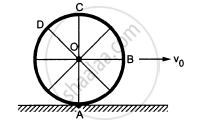MCQ | Q 9 | Page 195

Two uniform solid spheres having unequal masses and unequal radii are released from rest from the same height on a rough incline. If the spheres roll without slipping, ___________ .

• the heavier sphere reaches the bottom first

• the bigger sphere reaches the bottom first

• the two spheres reach the bottom together

• the information given is not sufficient to tell which sphere will reach the bottom first

MCQ | Q 10 | Page 195

A hollow sphere and a solid sphere having same mss and same radii are rolled down a rough inclined plane.

• The hollow sphere reaches the bottom first.

• The solid sphere reaches the bottom with greater speed

• The solid sphere reaches the bottom with greater kinetic energy.

• The two spheres will reach the bottom with same linear momentum.

MCQ | Q 11 | Page 195

A sphere cannot roll on

• a smooth horizontal surface

• a smooth inclined surface

• a rough horizontal surface

• a rough inclined surface.

MCQ | Q 12 | Page 195

In rear-wheel drive cars, the engine rotates the rear wheels and the front wheels rotate only because the car moves. If such a car accelerates on a horizontal road the friction

(a) on the rear wheels is in the forward direction

(b) on the front wheels is in the backward direction

(c) on the rear wheels has larger magnitude than the friction on the front wheels

(d) on the car is in the backward direction.

MCQ | Q 13 | Page 195

A sphere can roll on a surface inclined at an angle θ if the friction coefficient is more than $\frac{2}{7}g \tan\theta.$ Suppose the friction coefficient is $\frac{1}{7}g\ tan\theta.$ If a sphere is released from rest on the incline, _____________ .

• it will stay at rest

• it will make pure translational motion

• it will translate and rotate about the centre

• the angular momentum of the sphere about its centre will remain constant

MCQ | Q 14 | Page 195

A sphere is rolled on a rough horizontal surface. If gradually slows down and stops. The force of friction tries to

(a) decrease the linear velocity

(b) increase the angular velocity

(c) increase the linear momentum

(d) decrease the angular velocity.

MCQ | Q 15 | Page 195

The following figure shows a smooth inclined plane fixed in a car accelerating on a horizontal road. The angle of incline θ is related to the acceleration a of the car as a = g tanθ. If the sphere is set in pure rolling on the incline, _____________.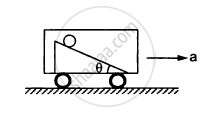• it will continue pure rolling

• it will slip down the plane

• its linear velocity will increase

• its linear velocity will slowly decrease

### HC Verma solutions for Class 12 Concepts of Physics 1 Chapter 10 Rotational Mechanics [Pages 195 - 200]

Q 1 | Page 195

A wheel is making revolutions about its axis with uniform angular acceleration. Starting from rest, it reaches 100 rev/sec in 4 seconds. Find the angular acceleration. Find the angle rotated during these four seconds.

Q 2 | Page 195

A wheel rotating with uniform angular acceleration covers 50 revolutions in the first five seconds after the start. Find the angular acceleration and the angular velocity at the end of five seconds.

Q 3 | Page 195

A wheel starting from rest is uniformly accelerated at 4 rad/s2 for 10 seconds. It is allowed to rotate uniformly for the next 10 seconds and is finally brought to rest in the next 10 seconds. Find the total angle rotated by the wheel.

Q 4 | Page 196

A body rotates about a fixed axis with an angular acceleration of one radian/second. Through what angle does it rotate during the time in which its angular velocity increases from 5 rad/s to 15 rad/s.

Q 5 | Page 196

Find the angular velocity of a body rotating with an acceleration of 2 rev/s2 as it completes the 5th revolution after the start.

Q 6.1 | Page 196

A disc of radius 10 cm is rotating about its axis at an angular speed of 20 rad/s. Find the linear speed of a point on the rim.

Q 6.2 | Page 196

A disc of radius 10 cm is rotating about its axis at an angular speed of 20 rad/s. Find the linear speed of the middle point of a radius.

Q 7 | Page 196

A disc rotates about its axis with a constant angular acceleration of 4 rad/s2. Find the radial and tangential accelerations of a particle at a distance of 1 cm from the axis at the end of the first second after the disc starts rotating.

Q 8 | Page 196

A block hangs from a string wrapped on a disc of radius 20 cm free to rotate about its axis which is fixed in a horizontal position. If the angular speed of the disc is 10 rad/s at some instant, with what speed is the block going down at that instant?

Q 9.1 | Page 196

Three particles, each of mass 200 g, are kept at the corners of an equilateral triangle of side 10 cm. Find the moment of inertial of the system about an axis joining two of the particles.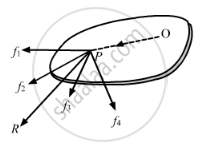Q 9.2 | Page 196

Three particles, each of mass 200 g, are kept at the corners of an equilateral triangle of side 10 cm. Find the moment of inertial of the system about an axis passing through one of the particles and perpendicular to the plane of the particles.Q 10 | Page 196

Particles of masses 1 g, 2 g, 3 g, .........., 100 g are kept at the marks 1 cm, 2 cm, 3 cm, ..........., 100 cm respectively on a metre scale. Find the moment of inertia of the system of particles about a perpendicular bisector of the metre scale.

Q 11 | Page 196

Find the moment of inertia of a pair of spheres, each having a mass mass m and radius r, kept in contact about the tangent passing through the point of contact.

Q 12 | Page 196

The moment of inertia of a uniform rod of mass 0⋅50 kg and length 1 m is 0⋅10 kg-m2about a line perpendicular to the rod. Find the distance of this line from the middle point of the rod.

Q 13 | Page 196

Find the radius of gyration of circular ring of radius r about a line perpendicular to the plane of the ring and passing through one of its particles.

Q 14 | Page 196

The radius of gyration of a uniform disc about a line perpendicular to the disc equals its radius. Find the distance of the line from the centre.

Q 15 | Page 196

Find the moment of inertia of a uniform square plate of mass m and edge a about one of its diagonals.

Q 16 | Page 196

The surface density (mass/area) of a circular disc of radius a depends on the distance from the centre as [rholeft( r right) = A + Br.] Find its moment of inertia about the line perpendicular to the plane of the disc thorough its centre.

Q 17 | Page 196

A particle of mass m is projected with a speed u at an angle θ with the horizontal. Find the torque of the weight of the particle about the point of projection when the particle is at the highest point.

Q 18 | Page 196

A simple pendulum of length l is pulled aside to make an angle θ with the vertical. Find the magnitude of the torque of the weight ω of the bob about the point of suspension. When is the torque zero?

Q 19 | Page 196

When a force of 6⋅0 N is exerted at 30° to a wrench at a distance of 8 cm from the nut it is just able to loosen the nut. What force F would be sufficient to loosen it if it acts perpendicularly to the wrench at 16 cm from the nut?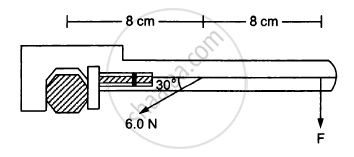Q 20 | Page 196

Calculate the total torque acting on the body shown in the following figure about the point O.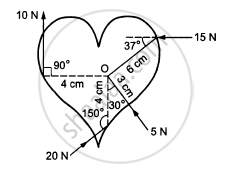Q 21 | Page 196

A cubical block of mass m and edge a slides down a rough inclined plane of inclination θ with a uniform speed. Find the torque of the normal force acting on the block about its centre.

Q 22 | Page 196

A rod of mass m and length L, lying horizontally, is free to rotate about a vertical axis through its centre. A horizontal force of constant magnitude F acts on the rod at a distance of L/4 from the centre. The force is always perpendicular to the rod. Find the angle rotated by the rod during the time t after the motion starts.

Q 23 | Page 196

A square plate of mass 120 g and edge 5⋅0 cm rotates about one of the edges. If it has a uniform angular acceleration of 0⋅2 rad/s2 what torque acts on the plate?

Q 24 | Page 196

Calculate the torque on the square plate of the previous problem if it rotates about a diagonal with the same angular acceleration.

Q 25 | Page 196

A flywheel of moment of inertia 5⋅0 kg-m2 is rotated at a speed of 60 rad/s. Because of the friction at the axle it comes to rest in 5⋅0 minutes. Find (a) the average torque of the friction (b) the total work done by the friction and (c) the angular momentum of the wheel 1 minute before it stops rotating.

Q 26 | Page 196

Because of the friction between the water in oceans with the earth's surface the rotational kinetic energy of the earth is continuously decreasing. If the earth's angular speed decreases by 0⋅0016 rad/day in 100 years find the average torque of the friction on the earth. Radius of the earth is 6400 km and its mass is 6⋅0 × 1024 kg.

Q 27 | Page 197

A wheel rotating at a speed of 600 rpm (revolutions per minute) about its axis is brought to rest by applying a constant torque for 10 seconds. Find the angular deceleration and the angular velocity 5 seconds after the application of the torque.

Q 28 | Page 197

A wheel of mass 10 kg and radius 20 cm is rotating at an angular speed of 100 rev/min when the motor is turned off. Neglecting the friction at the axle, calculate the force that must be applied tangentially to the wheel to bring it to rest in 10 revolutions.

Q 29 | Page 197

A cylinder rotating at an angular speed of 50 rev/s is brought in contact with an identical stationary cylinder. Because of the kinetic friction, torques act on the two cylinders accelerating the stationary one and decelerating the moving one. If the common magnitude of the acceleration and deceleration be one revolution per second square, how long will it take before the two cylinders have equal angular speed?

Q 30 | Page 197

A body rotating at 20 rad/s is acted upon by a constant torque providing it a deceleration of 2 rad/s2. At what time will the body have kinetic energy same as the initial value if the torque continues to act?

Q 31 | Page 197

A light rod of length 1 m is pivoted at its centre and two masses of 5 kg and 2 kg are hung from the ends as shown in the following figure. Find the initial angular acceleration of the rod assuming that it was horizontal in the beginning.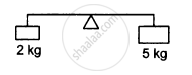Q 32 | Page 197

Suppose the rod in the previous problem has a mass of 1 kg distributed uniformly over its length.

(a) Find the initial angular acceleration of the rod.

(b) Find the tension in the supports to the blocks of mass 2 kg and 5 kg.

Q 33 | Page 197

The following figure shows two blocks of mass m and M connected by a string passing over a pulley. The horizontal table over which the mass m slides is smooth. The pulley has a radius r and moment of inertia I about its axis and it can freely rotate about this axis. Find the acceleration of the mass M assuming that the string does not slip on the pulley.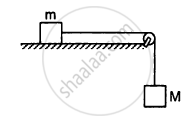Q 34 | Page 197

A string is wrapped on a wheel of moment of inertia 0⋅20 kg-m2 and radius 10 cm and goes through a light pulley to support a block of mass 2⋅0 kg as shown in the following figure. Find the acceleration of the block.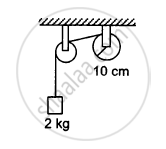Q 35 | Page 197

Suppose the smaller pulley of the previous problem has its radius 5⋅0 cm and moment of inertia 0⋅10 kg-m2. Find the tension in the part of the string joining the pulleys.

Q 36 | Page 197

The pulleys shown in the following figure are identical, each having a radius R and moment of inertia I. Find the acceleration of the block M.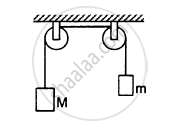Q 37 | Page 197

The descending pulley shown in the following figure has a radius 20 cm and moment of inertia 0⋅20 kg-m2. The fixed pulley is light and the horizontal plane frictionless. Find the acceleration of the block if its mass is 1⋅0 kg.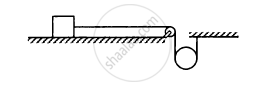Q 38 | Page 197

The pulley shown in the following figure has a radius 10 cm and moment of inertia 0⋅5 kg-m2about its axis. Assuming the inclined planes to be frictionless, calculate the acceleration of the 4⋅0 kg block.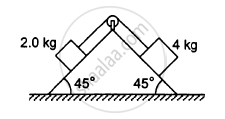Q 39 | Page 197

Solve the previous problem if the friction coefficient between the 2⋅0 kg block and the plane below it is 0⋅5 and the plane below the 4⋅0 kg block is frictionless.

Q 40 | Page 197

A uniform metre stick of mass 200 g is suspended from the ceiling thorough two vertical strings of equal lengths fixed at the ends. A small object of mass 20 g is placed on the stick at a distance of 70 cm from the left end. Find the tensions in the two strings.

Q 41 | Page 197

A uniform ladder of length 10⋅0 m and mass 16⋅0 kg is resting against a vertical wall making an angle of 37° with it. The vertical wall is frictionless but the ground is rough. An electrician weighing 60⋅0 kg climbs up the ladder. If he stays on the ladder at a point 8⋅00 m from the lower end, what will be the normal force and the force of friction on the ladder by the ground? What should be the minimum coefficient of friction for the electrician to work safely?

Q 42 | Page 198

Suppose the friction coefficient between the ground and
the ladder of the previous problem is 0.540. Find the
maximum weight of a mechanic who could go up and do
the work from the same position of the ladder.

Q 43 | Page 198

A 6⋅5 m long ladder rests against a vertical wall reaching a height of 6⋅0 m. A 60 kg man stands half way up the ladder. (a) Find the torque of the force exerted by the man on the ladder about the upper end of the ladder. (b) Assuming the weight of the ladder to be negligible as compared to the man and assuming the wall to be smooth, find the force exerted by the ground on the ladder.

Q 44 | Page 198

The door of an almirah is 6 ft high, 1⋅5 ft wide and weighs 8 kg. The door is supported by two hinges situated at a distance of 1 ft from the ends. If the magnitudes of the forces exerted by the hinges on the door are equal, find this magnitude.

Q 45 | Page 198

A uniform rod of length L rests against a smooth roller as shown in the following figure. Find the friction coefficient between the ground and the lower end if the minimum angle that the rod can make the horizontal is θ.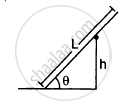Q 46 | Page 198

A uniform rod of mass 300 g and length 50 cm rotates at a uniform angular speed of 2 rad/s about an axis perpendicular to the rod through an end. Calculate (a) the angular momentum of the rod about the axis of rotation, (b) the speed of the centre of the rod and (c) its kinetic energy.

Q 47 | Page 198

A uniform square plate of mass 2⋅0 kg and edge 10 cm rotates about one of its diagonals under the action of a constant torque of 0⋅10 N-m. Calculate the angular momentum and the kinetic energy of the plate at the end of the fifth second after the start.

Q 48 | Page 198

Calculate the ratio of the angular momentum of the earth about its axis due to its spinning motion to that about the sun due to its orbital motion. Radius of the earth = 6400 km and radius of the orbit of the earth about the sun = 1⋅5 × 108 km.

Q 49 | Page 198

Two particles of masses m1 and m2 are joined by a light rigid rod of length r. The system rotates at an angular speed ω about an axis through the centre of mass of the system and perpendicular to the rod. Show that the angular momentum of the system is $L = \mu r^2 \omega$ where $\mu$ is the reduced mass of the system defined as $\mu = \frac{m_1 + m_2}{m_1 + m_2}$

Q 50 | Page 198

A dumb-bell consists of two identical small balls of mass 1/2 kg each connected to the two ends of a 50 cm long light rod. The dumb-bell is rotating about a fixed axis thorough the centre of the rod and perpendicular to it at an angular speed of 10 rad/s. An impulsive force of average magnitude 5⋅0 N acts on one of the masses in the direction of its velocity for 0⋅10 s. Find the new angular velocity of the system.

Q 51 | Page 198

A wheel of moment of inertia 0⋅500 kg-m2 and radius 20⋅0 cm is rotating about its axis at an angular speed of 20⋅0 rad/s. It picks up a stationary particle of mass 200 g at its edge. Find the new angular speed of the wheel.

Q 52 | Page 198

A diver having a moment of inertia of 6⋅0 kg-m2 about an axis thorough its centre of mass rotates at an angular speed of 2 rad/s about this axis. If he folds his hands and feet to decrease the moment of inertia to 5⋅0 kg-m2, what will be the new angular speed?

Q 53 | Page 198

A boy is seated in a revolving chair revolving at an angular speed of 120 revolutions per minute. Two heavy balls form part of the revolving system and the boy can pull the balls closer to himself or may push them apart. If by pulling the balls closer, the boy decreases the moment of inertia of the system from 6 kg-m2 to 2 kg-m2, what will be the new angular speed?

Q 54 | Page 198

A boy is standing on a platform which is free to rotate about its axis. The boy holds an open umbrella in his hands. The axis of the umbrella coincides with that of the platform. The moment of inertia of "the platform plus the boy system" is 3⋅0 × 10−3 kg-m2 and that of the umbrella is 2⋅0 × 10−3 kg-m2. The boy starts spinning the umbrella about the axis at an angular speed of 2⋅0 rev/s with respect to himself. Find the angular velocity imparted to the platform.

Q 55 | Page 198

A wheel of moment of inertia 0⋅10 kg-m2 is rotating about a shaft at an angular speed of 160 rev/minute. A second wheel is set into rotation at 300 rev/minute and is coupled to the same shaft so that both the wheels finally rotate with a common angular speed of 200 rev/minute. Find the moment of inertia of the second wheel.

Q 56 | Page 198

A kid of mass M stands at the edge of a platform of radius R which can be freely rotated about its axis. The moment of inertia of the platform is I. The system is at rest when a friend throws a ball of mass m and the kid catches it. If the velocity of the ball is $\nu$ horizontally along the tangent to the edge of the platform when it was caught by the kid, find the angular speed of the platform after the event.

Q 57 | Page 198

Suppose the platform of the previous problem is brought to rest with the ball in the hand of the kid standing on the rim. The kid throws the ball horizontally to his friend in a direction tangential to the rim with a speed $\nu$ as seen by his friend. Find the angular velocity with which the platform will start rotating.

Q 58 | Page 198

Suppose the platform with the kid in the previous problem is rotating in anticlockwise direction at an angular speed ω. The kid starts walking along the rim with a speed $\nu$ relative to the platform also in the anticlockwise direction. Find the new angular speed of the platform.

Q 59 | Page 199

A uniform rod of mass m and length l is struck at an end by a force F perpendicular to the rod for a short time interval t. Calculate (a) the speed of the centre of mass, (b) the angular speed of the rod about the centre of mass, (c) the kinetic energy of the rod and (d) the angular momentum of the rod about the centre of mass after the force has stopped to act. Assume that t is so small that the rod does not appreciably change its direction while the force acts.

Q 60 | Page 199

A uniform rod of length L lies on a smooth horizontal table. A particle moving on the table strikes the rod perpendicularly at an end and stops. Find the distance travelled by the centre of the rod by the time it turns through a right angle. Show that if the mass of the rod is four times that of the particle, the collision is elastic.

Q 61 | Page 199

Suppose the particle of the previous problem has a mass m and a speed $\nu$ before the collision and it sticks to the rod after the collision. The rod has a mass M. (a) Find the velocity of the centre of mass C of the system constituting "the rod plus the particle". (b) Find the velocity of the particle with respect to C before the collision. (c) Find the velocity of the rod with respect to C before the collision. (d) Find the angular momentum of the particle and of the rod about the centre of mass C before the collision. (e) Find the moment of inertia of the system about the vertical axis through the centre of mass C after the collision. (f) Find the velocity of the centre of mass C and the angular velocity of the system about the centre of mass after the collision.

Q 62 | Page 199

Two small balls A and B, each of mass m, are joined rigidly by a light horizontal rod of length L. The rod is clamped at the centre in such a way that it can rotate freely about a vertical axis through its centre. The system is rotated with an angular speed ω about the axis. A particle P of mass m kept at rest sticks to the ball A as the ball collides with it. Find the new angular speed of the rod.

Q 63 | Page 199

Two small balls A and B, each of mass m, are joined rigidly to the ends of a light rod of length L (see the following figure). The system translates on a frictionless horizontal surface with a velocity $\nu_0$ in a direction perpendicular to the rod. A particle P of mass m kept at rest on the surface sticks to the ball A as the ball collides with it. Find
(a) the linear speeds of the balls A and B after the collision, (b) the velocity of the centre of mass C of the system A + B + P and (c) the angular speed of the system about C after the collision.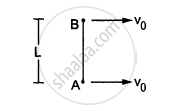[Hint : The light rod will exert a force on the ball B
only along its length.]

Q 64 | Page 199

Suppose the rod with the balls A and B of the previous problem is clamped at the centre in such a way that it can rotate freely about a horizontal axis through the clamp. The system is kept at rest in the horizontal position. A particle P of the same mass m is dropped from a height h on the ball B. The particle collides with B and sticks to it. (a) Find the angular momentum and the angular speed of the system just after the collision. (b) What should be the minimum value of h so that the system makes a full rotation after the collision.

Q 65 | Page 199

Two blocks of masses 400 g and 200 g are connected through a light string going over a pulley which is free to rotate about its axis. The pulley has a moment of inertia $1 \cdot 6 \times {10}^{- 4} kg - m^2$ and a radius 2⋅0 cm, Find (a) the kinetic energy of the system as the 400 g block falls through 50 cm, (b) the speed of the blocks at this instant.

Q 66 | Page 199

The pulley shown in the following figure has a radius of 20 cm and moment of inertia 0⋅2 kg-m2. The string going over it is attached at one end to a vertical spring of spring constant 50 N/m fixed from below, and supports a 1 kg mass at the other end. The system is released from rest with the spring at its natural length. Find the speed of the block when it has descended through 10 cm. Take g = 10 m/s2.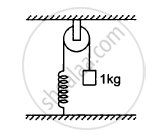Q 67 | Page 199

A metre stick is held vertically with one end on a rough horizontal floor. It is gently allowed to fall on the floor. Assuming that the end at the floor does not slip, find the angular speed of the rod when it hits the floor.

Q 68 | Page 199

A metre stick weighing 240 g is pivoted at its upper end in such a way that it can freely rotate in a vertical place through this end (see the following figure). A particle of mass 100 g is attached to the upper end of the stick through a light string of length 1 m. Initially, the rod is kept vertical and the string horizontal when the system is released from rest. The particle collides with the lower end of the stick and sticks there. Find the maximum angle through which the stick will rise.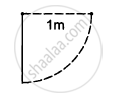Q 69 | Page 199

A uniform rod pivoted at its upper end hangs vertically. It is displaced through an angle of 60° and then released. Find the magnitude of the force acting on a particle of mass dm at the tip of the rod when the rod makes an angle of 37° with the vertical.

Q 70 | Page 199

A cylinder rolls on a horizontal place surface. If the speed of the centre is 25 m/s, what is the speed of the highest point?

Q 71 | Page 200

A sphere of mass m rolls on a plane surface. Find its kinetic energy at an instant when its centre moves with speed $\nu.$

Q 72 | Page 200

A string is wrapped over the edge of a uniform disc and the free end is fixed with the ceiling. The disc moves down, unwinding the string. Find the downward acceleration of the disc.

Q 73 | Page 200

A small spherical ball is released from a point at a height h on a rough track shown in the following figure. Assuming that it does not slip anywhere, find its linear speed when it rolls on the horizontal part of the track.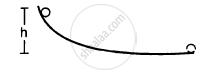Q 74 | Page 200

A small disc is set rolling with a speed $\nu$ on the horizontal part of the track of the previous problem from right to left. To what height will it climb up the curved part?

Q 75 | Page 200

A sphere starts rolling down an incline of inclination θ. Find the speed of its centre when it has covered a distance l.

Q 76 | Page 200

A hollow sphere is released from the top of an inclined plane of inclination θ. (a) What should be the minimum coefficient of friction between the sphere and the plane to prevent sliding? (b) Find the kinetic energy of the ball as it moves down a length l on the incline if the friction coefficient is half the value calculated in part (a).

Q 77 | Page 200

A solid sphere of mass m is released from rest from the rim of a hemispherical cup so that it rolls along the surface. If the rim of the hemisphere is kept horizontal, find the normal force exerted by the cup on the ball when the ball reaches the bottom of the cup.

Q 78 | Page 200

The following figure shows a rough track, a portion of which is in the form of a cylinder of radius R. With what minimum linear speed should a sphere of radius r be set rolling on the horizontal part so that it completely goes round the circle on the cylindrical part.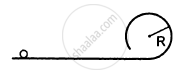Q 79 | Page 200

The following figure shows a small spherical ball of mass m rolling down the loop track. The ball is released on the linear portion at a vertical height H from the lowest point. The circular part shown has a radius R.
(a) Find the kinetic energy of the ball when it is at a point A where the radius makes an angle θ with the horizontal.
(b) Find the radial and the tangential accelerations of the centre when the ball is at A.
(c) Find the normal force and the frictional force acting on the if ball if H = 60 cm, R = 10 cm, θ = 0 and m = 70 g.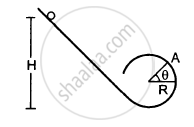Q 80 | Page 200

A thin spherical shell of radius R lying on a rough horizontal surface is hit sharply and horizontally by a cue. Where should it be hit so that the shell does not slip on the surface?

Q 81 | Page 200

A uniform wheel of radius R is set into rotation about its axis at an angular speed ω. This rotating wheel is now placed on a rough horizontal surface with its axis horizontal. Because of friction at the contact, the wheel accelerates forward and its rotation decelerates till the wheel starts pure rolling on the surface. Find the linear speed of the wheel after it starts pure rolling.

Q 82 | Page 200

A thin spherical shell lying on a rough horizontal surface is hits by a cue in such a way that the line of action passes through the centre of the shell. As a result, the shell starts moving with a linear speed $\nu$ without any initial angular velocity. Find the linear speed of the shell after it starts pure rolling on the surface.

Q 83 | Page 200

A hollow sphere of radius R lies on a smooth horizontal surface. It is pulled by a horizontal force acting tangentially from the highest point. Find the distance travelled by the sphere during the time it makes one full rotation.

Q 84 | Page 200

A solid sphere of mass 0⋅50 kg is kept on a horizontal surface. The coefficient of static friction between the surfaces in contact is 2/7. What maximum force can be applied at the highest point in the horizontal direction so that the sphere does not slip on the surface?

Q 85 | Page 200

A solid sphere is set into motion on a rough horizontal surface with a linear speed ν in the forward direction and an angular speed ν/R in the anticlockwise directions as shown in the following figure. Find the linear speed of the sphere (a) when it stops rotating and (b) when slipping finally ceases and pure rolling starts.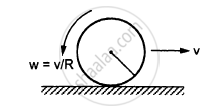Q 86 | Page 200

A solid sphere rolling on a rough horizontal surface with a linear speed ν collides elastically with a fixed, smooth, vertical wall. Find the speed of the sphere after it has started pure rolling in the backward direction.

## Chapter 10: Rotational Mechanics## HC Verma solutions for Class 12 Concepts of Physics 1 chapter 10 - Rotational Mechanics

HC Verma solutions for Class 12 Concepts of Physics 1 chapter 10 (Rotational Mechanics) include all questions with solution and detail explanation. This will clear students doubts about any question and improve application skills while preparing for board exams. The detailed, step-by-step solutions will help you understand the concepts better and clear your confusions, if any. Shaalaa.com has the CBSE Class 12 Concepts of Physics 1 solutions in a manner that help students grasp basic concepts better and faster.

Further, we at Shaalaa.com provide such solutions so that students can prepare for written exams. HC Verma textbook solutions can be a core help for self-study and acts as a perfect self-help guidance for students.

Concepts covered in Class 12 Concepts of Physics 1 chapter 10 Rotational Mechanics are Dynamics of Rotational Motion About a Fixed Axis, Angular Momentum in Case of Rotation About a Fixed Axis, Rolling Motion, Momentum Conservation and Centre of Mass Motion, Centre of Mass of a Rigid Body, Centre of Mass of a Uniform Rod, Rigid Body Rotation, Equilibrium of Rigid Bodies, Moment of Inertia, Theorems of Perpendicular and Parallel Axes, Kinematics of Rotational Motion About a Fixed Axis, Motion - Rigid Body, Centre of Mass, Motion of Centre of Mass, Linear Momentum of a System of Particles, Vector Product of Two Vectors, Angular Velocity and Its Relation with Linear Velocity, Torque and Angular Momentum, Equations of Rotational Motion, Comparison of Linear and Rotational Motions, Values of Moments of Inertia for Simple Geometrical Objects (No Derivation).

Using HC Verma Class 12 solutions Rotational Mechanics exercise by students are an easy way to prepare for the exams, as they involve solutions arranged chapter-wise also page wise. The questions involved in HC Verma Solutions are important questions that can be asked in the final exam. Maximum students of CBSE Class 12 prefer HC Verma Textbook Solutions to score more in exam.

Get the free view of chapter 10 Rotational Mechanics Class 12 extra questions for Class 12 Concepts of Physics 1 and can use Shaalaa.com to keep it handy for your exam preparation